International
Tables for
Crystallography
Volume D
Physical properties of crystals
Edited by A. Authier

International Tables for Crystallography (2006). Vol. D, ch. 1.1, p. 24

## Section 1.1.4.10.1. Introduction

A. Authiera*

aInstitut de Minéralogie et de la Physique des Milieux Condensés, Bâtiment 7, 140 rue de Lourmel, 75015 Paris, France
Correspondence e-mail: aauthier@wanadoo.fr

#### 1.1.4.10.1. Introduction

| top | pdf |

Many tensors representing physical properties or physical quantities appear in relations involving symmetric tensors. Consider, for instance, the strainresulting from the application of an electric field E (the piezoelectric effect):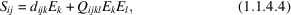where the first-order terms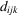represent the components of the third-rank converse piezoelectric tensor and the second-order terms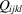represent the components of the fourth-rank electrostriction tensor. In a similar way, the direct piezoelectric effect corresponds to the appearance of an electric polarization P when a stress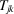is applied to a crystal: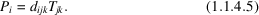Owing to the symmetry properties of the strain and stress tensors (see Sections 1.3.1and 1.3.2) and of the tensor product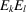, there occurs a further reduction of the number of independent components of the tensors which are engaged in a contracted product with them, as is shown in Section 1.1.4.10.3for third-rank tensors and in Section 1.1.4.10.5for fourth-rank tensors.